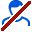Sold: 1 last one 27.03.2015
Refunds: 0

Content: 30716104308507.docx 39,47 kB## SellerИФЦ Студия information about the seller and his items

## Product description

Problem 1. Steam regression and correlation.

In areas of the region display data for 199X, the (see. Table

its variants).

Required:

1. A linear regression equation y pair of x.

2. Calculate the linear correlation coefficient, and

average error of approximation.

3. To assess the statistical significance of the regression parameters, and

Correlation using F-test and the Fisher Student t-test.

4. Run the forecast salary y in the forecast

meaning the average per capita subsistence level x, is 107%

on average.

5. Evaluate the accuracy of prediction by calculating prediction error and

confidence interval.

6. On the same graph to construct the original data and the theoretical

line.